Nisha Desai
5 ACT Info and Tips

Guide to Functions on the ACT Math Test + Practice Questions

Is your SAT score enough to get you into your dream school?

Our free chancing engine takes into consideration your SAT score, in addition to other profile factors, such as GPA and extracurriculars. Create a free account to discover your chances at hundreds of different schools.

What’s Covered:

On the ACT Math Test, functions are unavoidable. Whether you’re being tested directly on function knowledge or not, the majority of questions cover a fundamental understanding of functions. So, make sure you brush up on your function knowledge before taking the ACT Math Test! In this post, we’ll go over some of the important concepts you’ll need to know, as well as official practice questions that will help you assess your understanding.

Overview of the ACT Math Test

The ACT Math Test consists of 60 questions, to be answered in 60 minutes. These questions are designed to test your knowledge of various mathematical concepts. The questions fall into the following categories:

• Preparing for Higher Math
• Integrating Essential Skills
• Modeling

Questions involving functions on the ACT Math Test fall under the category Preparing for Higher Mathematics. About 12–15% (or 7–9 questions) of the test involves functions, so you’ll definitely need a comprehensive understanding of functions to do well.

Functions on the ACT + Practice Questions

Inputs and outputs

The most basic definition of a function is that it takes in a value and produces a result. However, this isn’t as simple as it looks. In fact, for every input, there can only be one output. This is also known as the Vertical Line Test:

Function: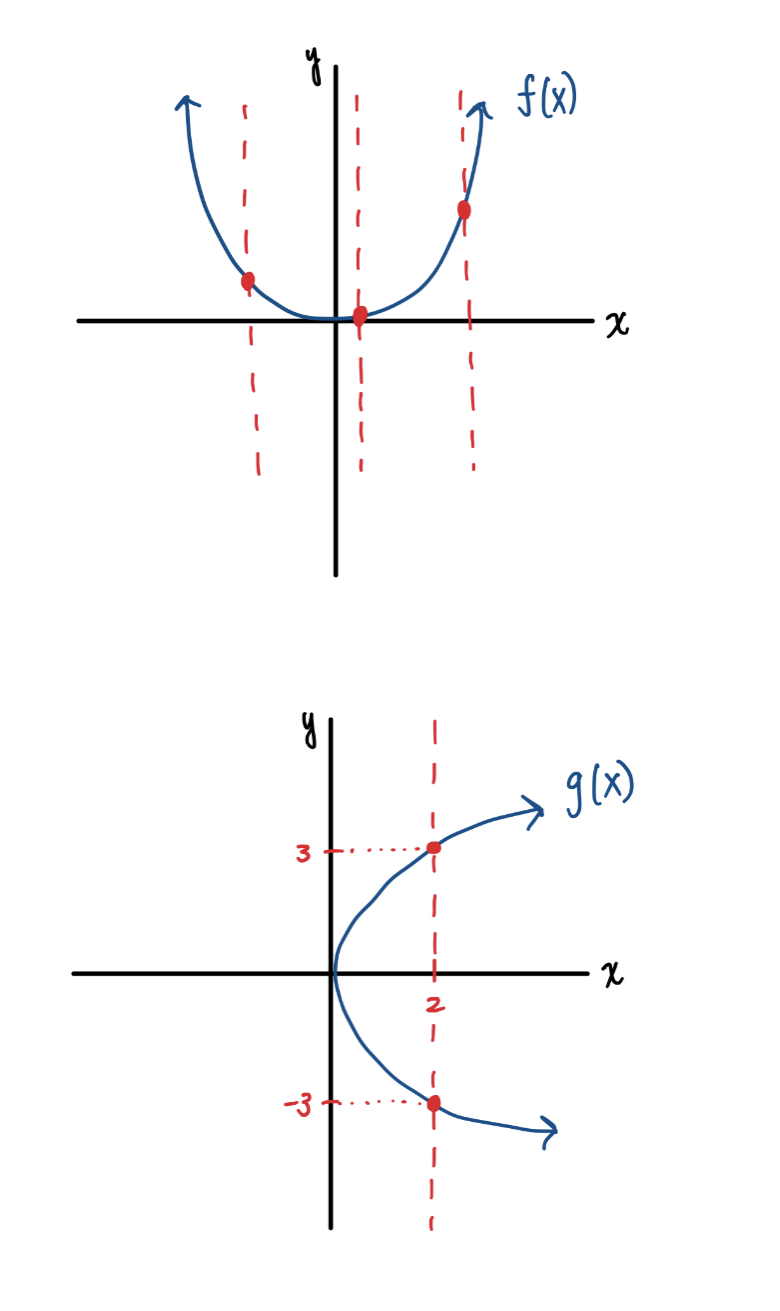In this case, $$f(x)$$ is a function since it passes the Vertical Line Test. That is, at any point on the graph, if you drew a vertical line (represented in red), you would only intersect the graph precisely once. This means that each input has been assigned at most one output, so we have a function.

NOT a function:On the other hand, $$g(x)$$ is not a function. This is because it does not pass the Vertical Line Test. At $$x=2$$, the function actually has two outputs: 3 and -3. As a result, this fails the condition that must be met in order for something to be considered a function.

Now that we have defined what a function is, let’s take a look at some practical applications. The following questions are taken from the ACT’s official practice tests.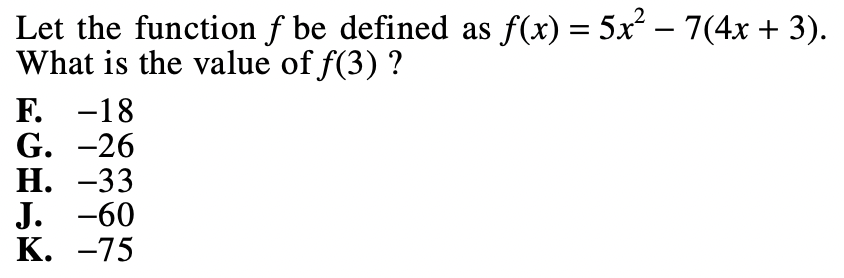This question is fairly simple. We are given a function and an input, and are asked to find the output. Let’s plug in our input into our function to determine the output. This means that anywhere we see an $$x$$, we replace it with a 3.

$$f(3)=5(3)^2-7(4(3)+3)$$

$$f(3)=45-7(15)$$

$$f(3)= -60$$

Let’s take a look at another problem regarding inputs and outputs. This time, the information is displayed graphically, rather than algebraically.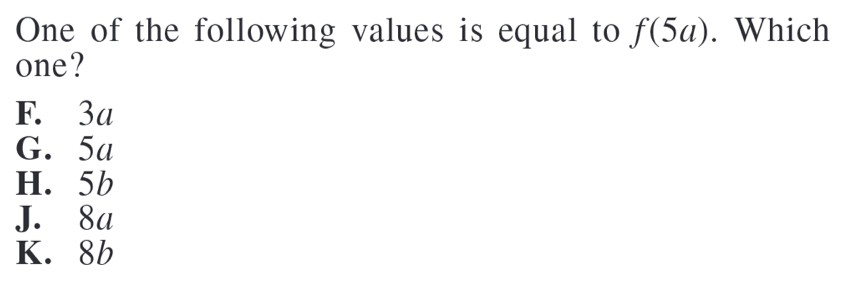To find $$f(5a)$$, we need to determine which output is associated with the input $$5a$$. Since the data is graphical, our inputs are $$x$$ values and our outputs are $$y$$ values. Let’s look at where $$x=5a$$: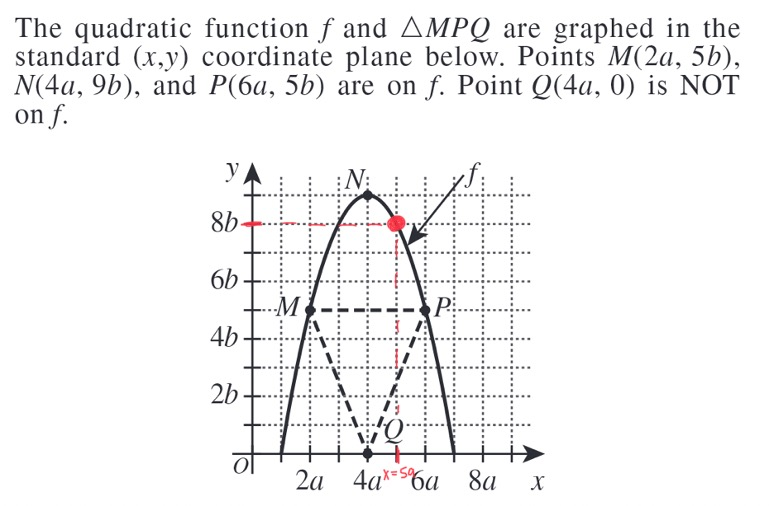At $$x=5a$$, we have that the function value is $$8b$$. So, the answer is option K.

Functions as models

Functions can also be used to represent real-world applications. This means you could come across functions in the context of word problems. Take a look at the following example: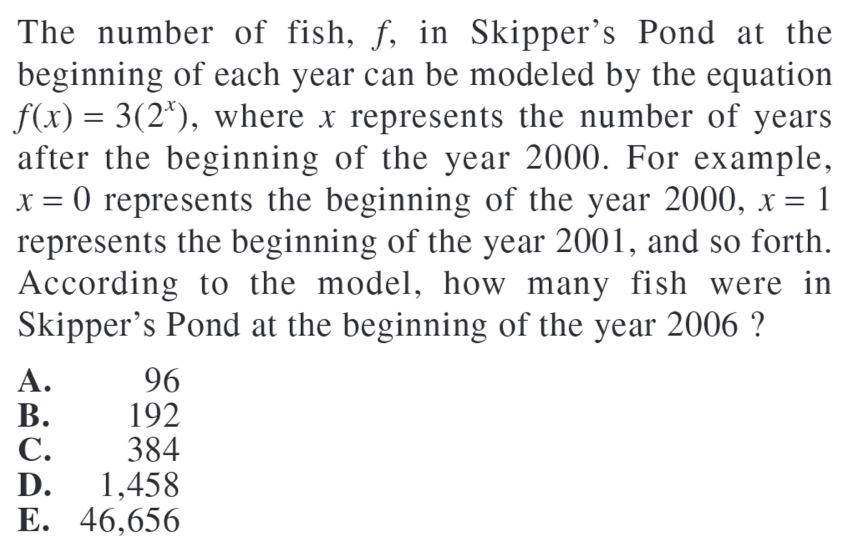The easiest way to solve word problems is to begin by separating the numerical information from the rest of the text. This allows you to focus on one thing at a time and makes the problem easier to solve. We’re given a function, $$f(x)=3(2^x)$$ and we’re asked to find the output that is associated with the year 2006. We’re told that $$x$$ represents the number of years after 2000. So, in 2006, $$x=6$$.

Now, we can plug our input into our function:

$$f(6)=3(2^6)=192$$ fish. So, our answer is B.

As part of our free guidance platform, our Admissions Assessment tells you what schools you need to improve your SAT score for and by how much. Sign up to get started today.

Trigonometric functions

Trigonometric functions are another type of function that you will be evaluated on during the ACT Math section. You must be aware of the fundamental features of each operation. This question is an example of what you may encounter on the actual test.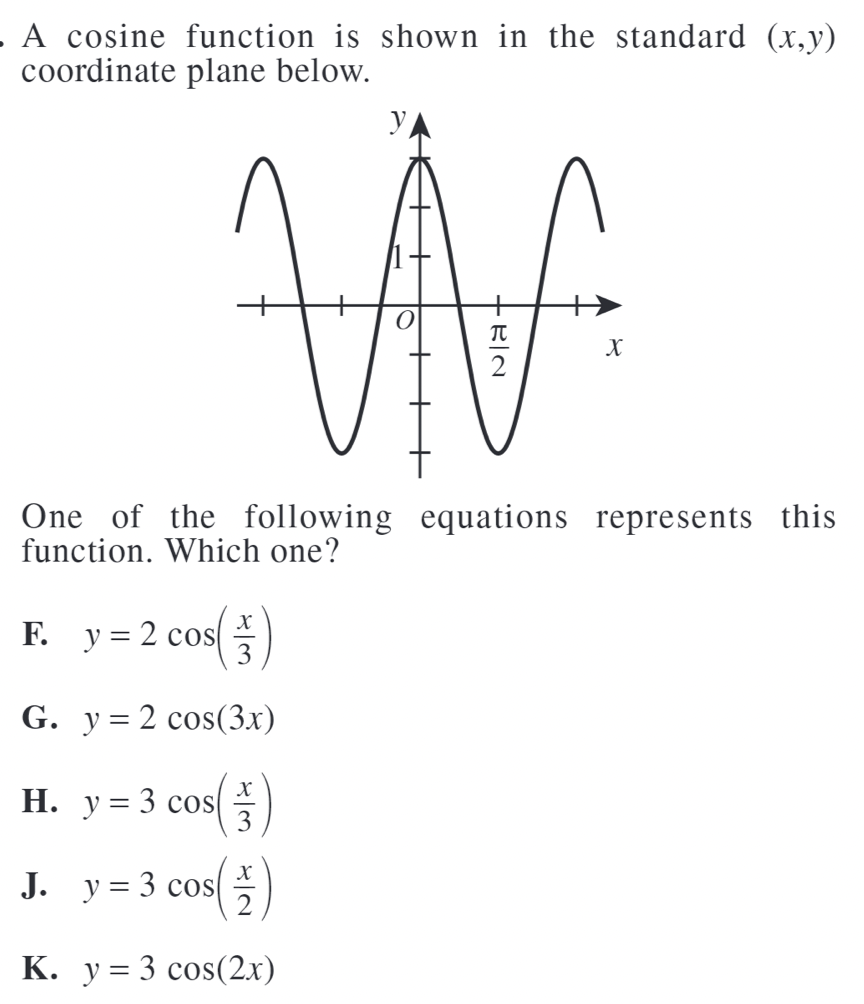To see which equation corresponds to our graph, we can simply pick a few significant points.

First, let’s arbitrarily choose $$(0,3)$$. Because our graph occurs at this point, it should also satisfy the equation. Let’s plug $$x=0$$ into each equation and see if it yields $$y=3$$.

F: $$y=2\cos{(\frac{0}{3})}=2$$

G: $$y=2\cos{(3\:\cdot\:0)}=2$$

H: $$y=3\cos{(\frac{0}{3})}=3$$

J: $$y=3\cos{(\frac{0}{2})}=3$$

K: $$y=3\cos{(2\:\cdot\:0)}=3$$

So, we see that answer choices F and G are incorrect.

Next, let’s pick another point. We can use $$(\pi/2,-3)$$. Let’s repeat the same process:

H: y=$$3\cos{(\frac{\frac{\pi}{2}}{3})}=3\frac{\sqrt{3}}{2}$$

J: y=$$3\cos{(\frac{\frac{\pi}{2}}{2})}=3\frac{\sqrt{2}}{2}$$

K: y=$$3\cos{(2\frac{\pi}{2})}= -3$$

Therefore, the only correct answer is K.

Composite functions

Composite functions might be a little difficult to understand at first. In essence, composite functions are made up of two or more distinct functions that operate together. This may appear confusing, but if we focus on one function at a time, composite functions become a lot simpler. Let’s put this information to use by working through an example one step at a time: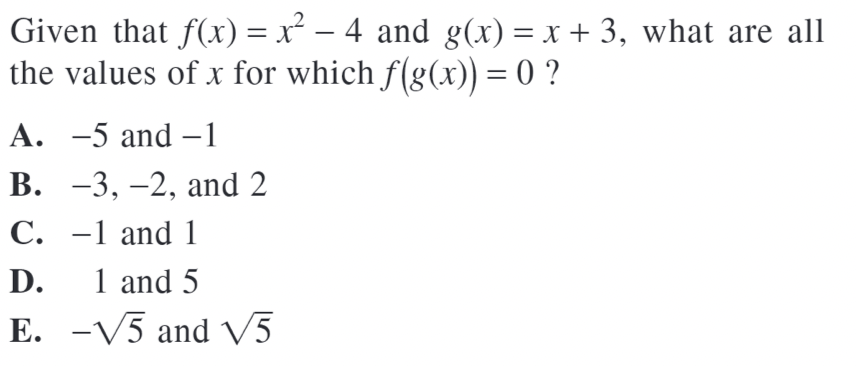This problem actually wants us to work backwards. They give us an output of $$0$$ and ask us to find the corresponding input.

We start with $$f(g(x))=0$$. Let’s ignore $$g(x)$$ for now and find the input that makes $$f(x)=0$$. So, we set up the following equation and solve for $$x$$:

$$x^2-4=0$$

$$(x+2)(x-2)=0$$

So, $$x=2$$ or $$x=-2$$. Then, we have that $$f(2)=0$$ and $$f(-2)=0$$.

So, we’re now trying to find $$x$$ such that $$g(x)=2$$ or $$g(x)=-2$$. Let’s complete the same process to solve for $$x$$.

First, let’s solve for $$g(x)=2$$:

$$x+3=2$$

$$x=-1$$

Next, let’s solve for $$g(x)=-2$$:

$$x+3=-2$$

$$x=-5$$

This means that for $$f(g(x))=0$$, we can have $$x=-1$$ or $$x=-5$$, which corresponds to answer choice A.

How Will My ACT Score Impact My College Chances?

Colleges use a metric called the Academic Index (AI) to assess the academic strength of your application. Your AI is calculated by taking into account statistics like your GPA and standardized test scores, including the ACT. Some highly selective schools will even automatically reject applicants whose AIs are too low (though this has changed in the past few cycles in light of COVID-19).

Still, having a competitive ACT score is important to improve your chances at any school. To see how your score affects your chances, you can use CollegeVine’s free Admissions Calculator. This tool considers your GPA, test scores, extracurriculars, and more to determine your unique chances at the schools of your choice. It’ll even offer tips on how to improve other aspects of your profile!

Check out some of these additional resources for the ACT Math Test:Short Bio
Nisha Desai is a second year student at the University of California, Riverside. She recently started working at CollegeVine, but has done application guidance and tutoring in a private capacity for a couple of years. She is in school to eventually get her Masters in Education and enjoys reading and running in her free time.

Don't miss out on the best high school & college admissions resources!

Join thousands of students and parents getting exclusive high school, test prep, and college admissions information.